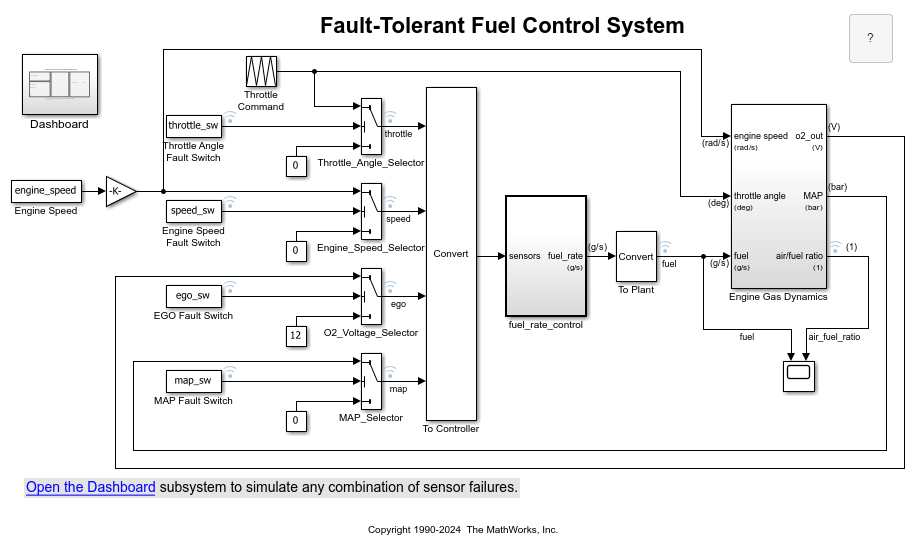# LUTCompressionResult

Optimized lookup table data for all Lookup Table blocks in a system

Since R2020a

## Description

A `LUTCompressionResult` object contains the optimized lookup table data for all Lookup Table blocks in a system. To create a `LUTCompressionResult` object, use the `FunctionApproximation.compressLookupTables` function. To replace the lookup tables in your system with the optimized version, use the `replace` function.

## Creation

Create a `LUTCompressionResult` object using `FunctionApproximation.compressLookupTables`.

## Properties

expand all

Units for `MaxMemoryUsage` property, specified as `'bits'`, `'bytes'`, or one of the other enumerated options.

Data Types: `char`

Table summarizing the effects of compression. The table contains one row for each lookup table compressed in the system and its corresponding memory savings.

Data Types: `table`

Number of lookup tables found in the specified system, specified as an integer-valued scalar.

Data Types: `double`

Number of lookup tables compressed in the system, specified as an integer-valued scalar.

Data Types: `double`

Total memory of all lookup tables in the system before compression, returned as a scalar. You can specify the units of this property by using the `MemoryUnits` property.

Data Types: `double`

Total memory of all lookup tables in the system after compression, returned as a scalar. You can specify the units of this property by using the `MemoryUnits` property.

Data Types: `double`

Difference between the total memory of all lookup tables in the system before and after compression, returned as a scalar. You can specify the units of this property by using the `MemoryUnits` property.

Data Types: `double`

Percentage reduction in the memory used by the lookup tables in the system after compression, returned as a scalar.

Data Types: `double`

System containing compressed lookup tables, returned as a character vector. `SUD` is the same as the `system` input argument of the `FunctionApproximation.compressLookupTables` function.

Data Types: `char`

Word lengths used for breakpoints and table data in the compressed lookup tables, returned as a scalar or vector of integers.

Data Types: `double`

`Simulink.FindOptions` object specifying options for finding lookup tables in the system.

## Object Functions

 `replace` Replace all Lookup Table blocks with compressed lookup tables `revert` Revert compressed Lookup Table blocks to original versions

## Examples

collapse all

This example shows how to compress all Lookup Table blocks in a system.

Open the model containing the lookup tables that you want to compress.

```system = 'sldemo_fuelsys'; open_system(system) ```Use the `FunctionApproximation.compressLookupTables` function to compress all of the lookup tables in the model. The output specifies all blocks that are modified and the memory savings for each.

```compressionResult = FunctionApproximation.compressLookupTables(system) ```
```- Found 5 supported lookup tables - Percent reduction in memory for compressed solution - 2.37% for sldemo_fuelsys/fuel_rate_control/airflow_calc/Pumping Constant - 2.37% for sldemo_fuelsys/fuel_rate_control/control_logic/Throttle.throttle_estimate/Throttle Estimation - 3.55% for sldemo_fuelsys/fuel_rate_control/control_logic/Speed.speed_estimate/Speed Estimation - 6.38% for sldemo_fuelsys/fuel_rate_control/control_logic/Pressure.map_estimate/Pressure Estimation - 9.38% for sldemo_fuelsys/fuel_rate_control/airflow_calc/Ramp Rate Ki compressionResult = LUTCompressionResult with properties: MemoryUnits: bytes MemoryUsageTable: [5x5 table] NumLUTsFound: 5 NumImprovements: 5 TotalMemoryUsed: 6024 TotalMemoryUsedNew: 5796 TotalMemorySavings: 228 TotalMemorySavingsPercent: 3.7849 SUD: 'sldemo_fuelsys' WordLengths: [8 16 32] FindOptions: [1x1 Simulink.internal.FindOptions] Display: 1 ```

Use the `replace` function to replace each Lookup Table block with a block containing the original and compressed version of the lookup table.

```replace(compressionResult); ```

You can revert the lookup tables back to their original state using the `revert` function.

```revert(compressionResult); ```

## Version History

Introduced in R2020a